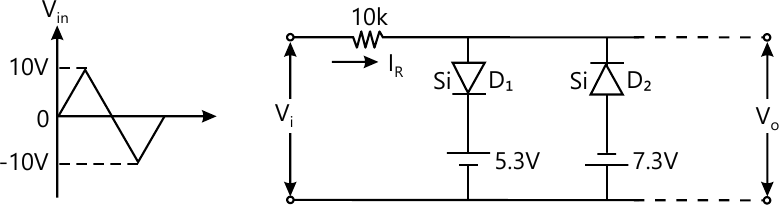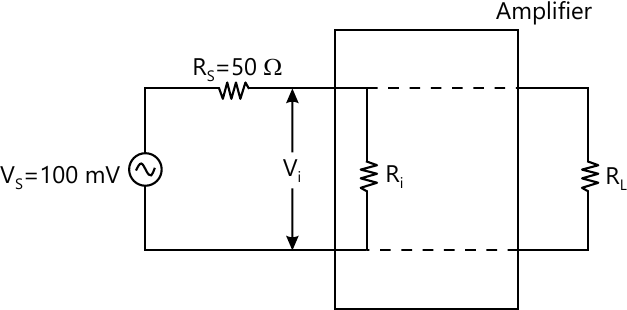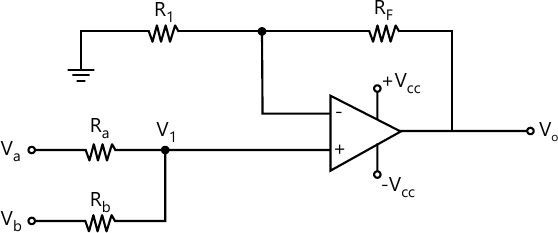MORE IN Basic Electronics Engineering (BXE)
SPPU First Year Engineering (Semester 2)
Basic Electronics Engineering (BXE)
April 2013
Total marks: --
Total time: --
INSTRUCTIONS
(1) Assume appropriate data and state your reasons
(2) Marks are given to the right of every question
(3) Draw neat diagrams wherever necessary

Answer any one question from Q1 & Q2
1 (a) Sketch IR and VO w. r .t time for the network shown in fig. A. Assume both the diodes are silicon type with Vf=0.7 V6 M
1 (b) For a BJT as a switch why CB and cc configurations are not preferred.
2 M
1 (c) Explain how Ri and Ro affect the performance of the BJT voltage amplifire.
4 M

2 (a) Explain with V-I characteristics the working of zener diode as voltage regulator.
6 M
2 (b) In the voltage amplifire shown in Fig B, Vs=100m V Rs=50 Ω
(i) Calculate input voltage Vi if the input resistance Ri is 600 Ω
(ii) What should be the value of Ri to get Vi=75 mV.6 M

Answer any one question from Q3 & Q4
3 (a) In fig. C if Va=+2V Vb=+4V
Ra=Rb=R1 = 1K Ω and Rf=3 k Ω determine the voltage V1 at non-inverting terminal of OP-AMP and out voltage V04 M
3 (b) Draw the block diagram of full adder using two half adder, explain its working with proper expression for sum and carry.
6 M
3 (c) Explain how EX-OR gate can be used as an invertor.
2 M

4 (a) With neat waveform explain IC555 in astable mode.
6 M
4 (b) Implement the following logic expression with minimum number of NAND gate
(i) y1=B(D +C D)
(ii) y2=AB+CD+B C
6 M

Answer any one question from Q5 & Q6
5 (a) Explain in detail, the selection criteria for transducer.
6 M
5 (b) Explain in detail
i) construction of TRIAC
ii) characteristics of TRIAC
iii) modes of oper Ation
7 M

6 (a) Explain with block diagram an electronic weighing machine.
6 M
6 (b) Explain the construction of DIAC w.r.t
i) Characteristics
ii) Application
7 M

7 (a) What is the importance of modulation index. Draw the AM waveform for
i) Liner modulation
ii) Over modulation
iii) Modulation index = 0
8 M
7 (b) Explain the basic structure of mobile phone system.
5 M

8 (a) With respect to FM explain
i) Frequency deviation
ii) Modulation index
iii) Deviation ratio
iv) Frequency spectrum of FM
8 M
8 (b) Write a note on co-axial cable and optical fibre cable.
5 M

More question papers from Basic Electronics Engineering (BXE)